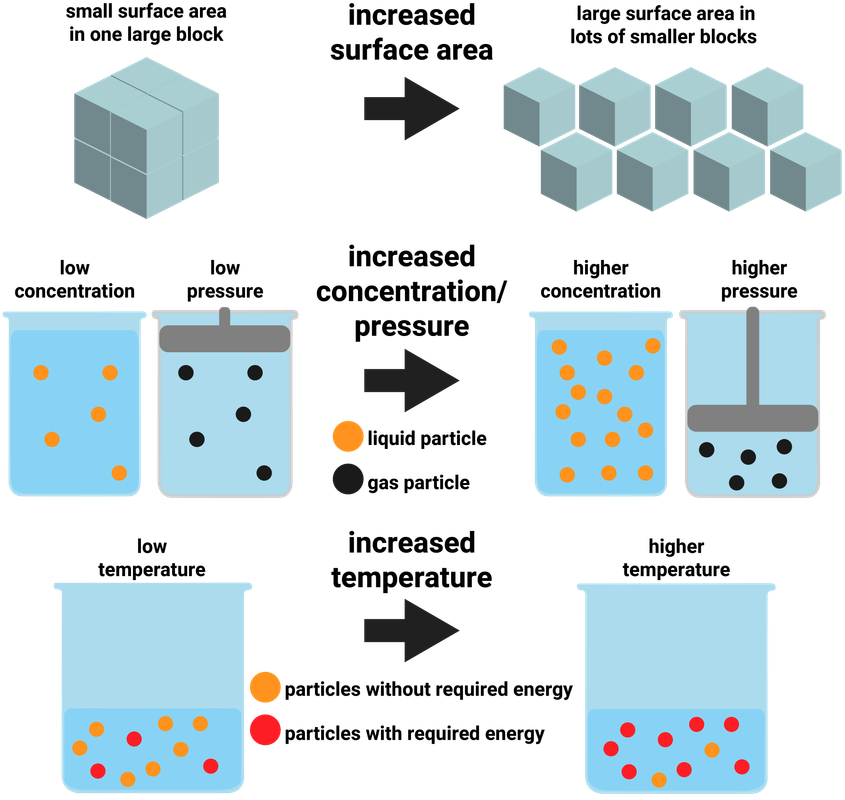# Rate of Reaction

### Collision Theory

Collision theory explains how various factors affect rates of reactions. According to this theory, chemical reactions can occur only when reacting particles collide with each other and with sufficient energy.

Not every collision is successful. The particles have to collide:

• at the right angle
• with the right amount of energy (the activation energy)

Increasing the concentration of reactants in solution, the pressure of reacting gases, and the surface area of solid reactants increases the frequency of collisions and so increases the rate of reaction.

Increasing the temperature increases the frequency of collisions and makes the collisions more energetic, and so increases the rate of reaction.### Calculating Rate

The shorter the reaction time is, the faster the rate of the reaction. We can calculate rate of reaction using one of three equations:

mean rate of reaction = quantity of reactant used ÷ time taken

or

mean rate of reaction = quantity of product made ÷ time taken​

or

mean rate of reaction = change in mass/volume ÷ change in time

The quantity of reactant or product can be measured by the mass in grams or by a volume in cm3.
The units of rate of reaction may be given as g/s or cm3/s.
For the Higher Tier, students are also required to use quantity of reactants in terms of moles and units for rate of reaction in mol/s.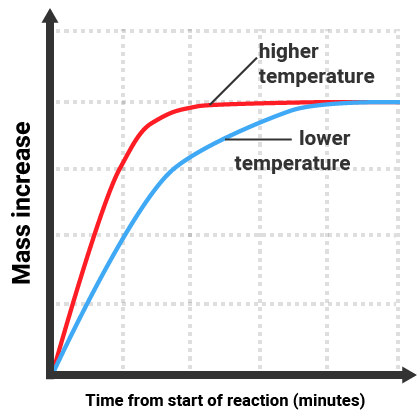The gradient of the line is equal to the rate of reaction. The reaction with the higher temperature:

• gives a steeper line (gradient)
• finishes sooner
• still produces the same mass increase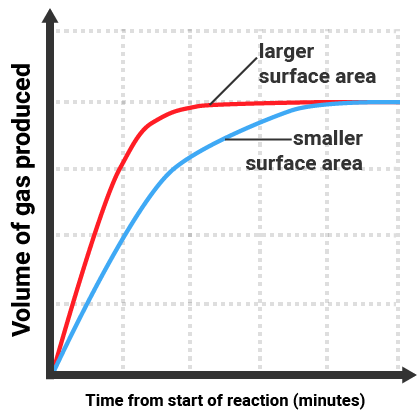The gradient of the line is equal to the rate of reaction. The reaction with the larger surface area:

• gives a steeper line (gradient)
• finishes sooner
• still produces the same volume increase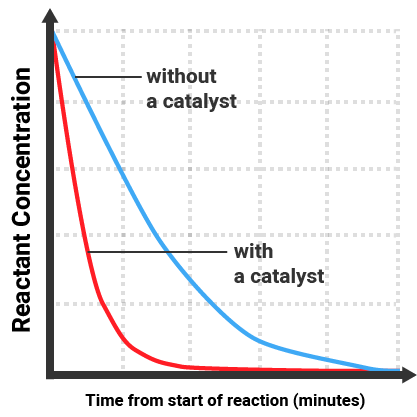The gradient of the line is equal to the rate of reaction. The reaction with a catalyst:

• gives a steeper line (gradient)
• finishes sooner
• still causes all reactant to be used up

### Measuring Rates

There are three main ways to measure the rate of a reaction:

1. the change of mass of reactants over time
2. the volume of gas produced over time
3. the time taken for a solution to change colour, or form a precipitate

### Mass change

This method works by measuring the amount of mass change during set time intervals, and then the data can be plotted on a graph.

### Volume of gas

Often easier than measuring small changes in mass. This method works by measuring the amount of gas collected during set time intervals, and then the data can be plotted on a graph.

### Colour change/precipitation

This can either be done to measure the change in colour, or to measure change in opaqueness (disappearing cross - as the cross slowly 'disappears' due to precipitate forming). The end point is quite inaccurate when using the human eye, so to increase accuracy we can use digital data loggers instead.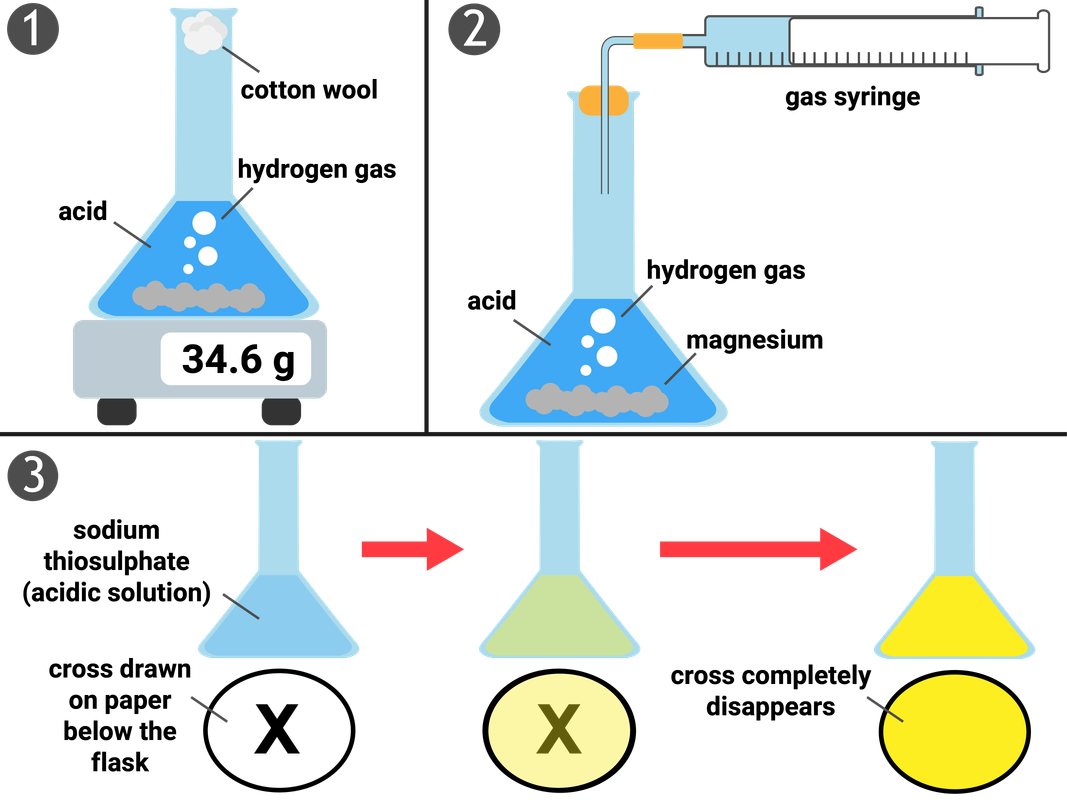### Temperature

Increasing the temperature of a reaction gives the particles more energy to collide. This leads to more frequent, successful collisions and so the rate of reaction increases.

### Concentration of solution, or Pressure of gas

Increasing the concentration (for liquids)/pressure (for gases) of reactants, increases the amount of particles in a given volume. This leads to more frequent, successful collisions and so the rate of reaction increases.

### Surface area of solid reactants

Increasing the surface area of reactants frees up more particles that are available to collide right away. This doesn't add more particles in to the reaction, it only allows particles that would have once had to wait for other particles to react first to be available. This leads to more frequent, successful collisions and so the rate of reaction increases.

### Catalysts

Catalysts change the rate of chemical reactions but are not used up during the reaction. Different reactions need different catalysts. Enzymes act as catalysts in biological systems.

Catalysts increase the rate of reaction by providing a different pathway for the reaction that has a lower activation energy.